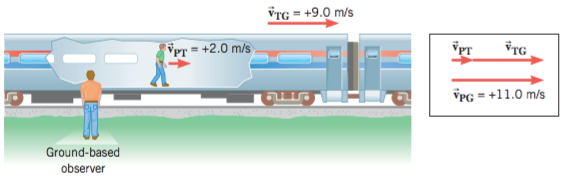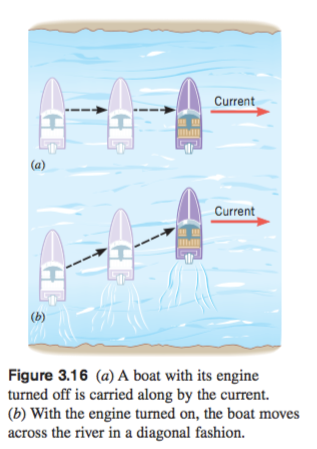top of page
Search

# Relative velocity - 相对速度V PT = velocity of the Passenger relative to the Train = + 2.0 m/s

V TG = velocity of the Train relative to the Ground = + 9.0 m/s

V PG = velocity of the Passenger relative to the Ground = + 11 m/s

V PG = V PT + V TG

Thus V PG = (2.0 m/s) + (9.0 m/s) = + 11 m/sV BW = velocity of the Boat relative to the water

V WS = velocity of the Water relative to the Shore

V BS = velocity of the Boat relative to the Shore

Thus V BS = V BW + V WS

#Physics

bottom of page